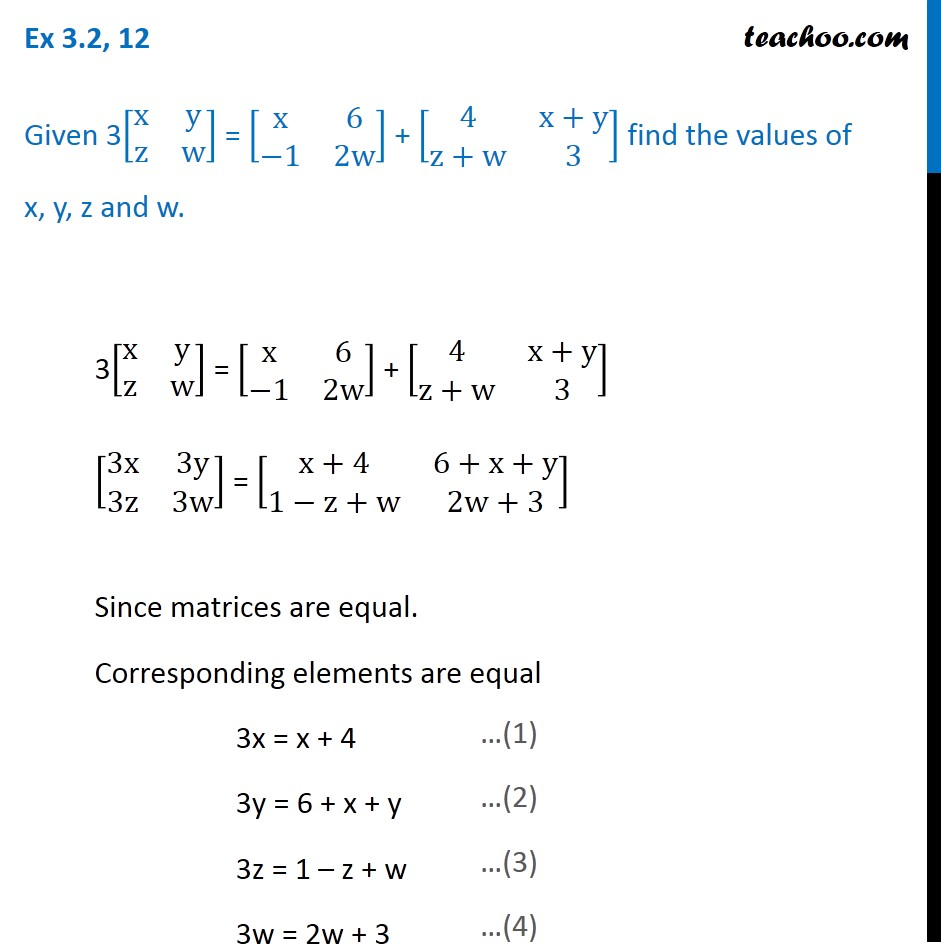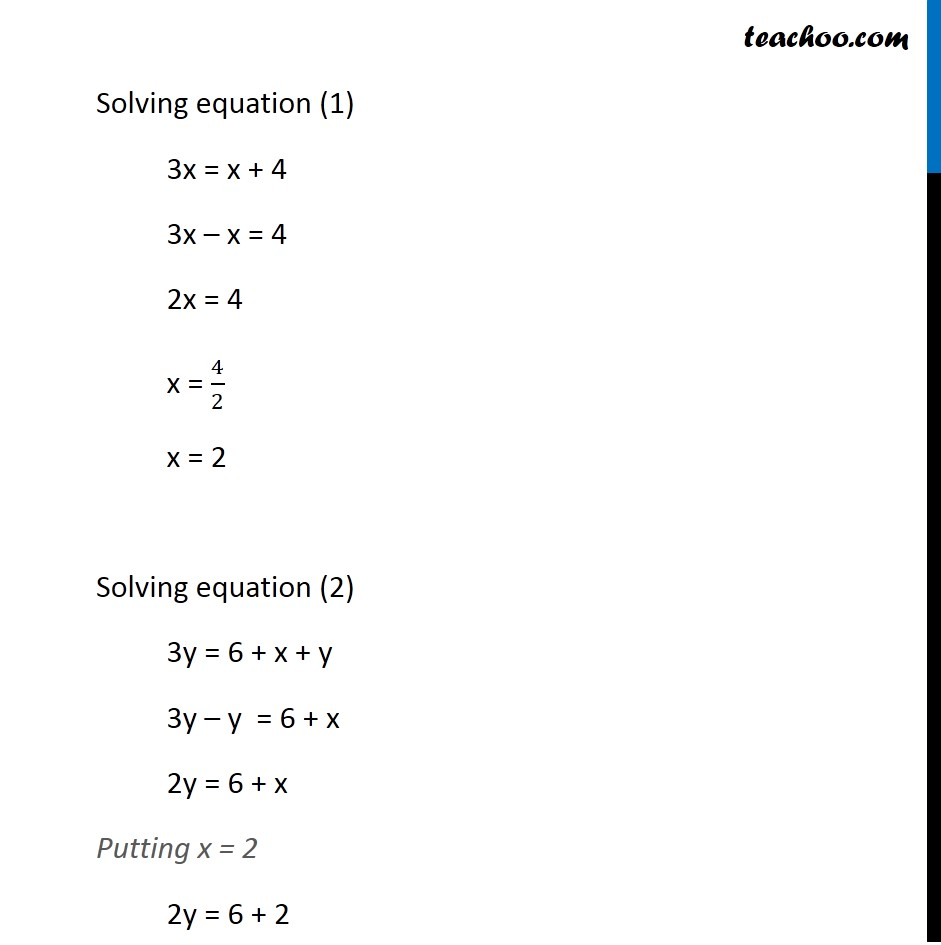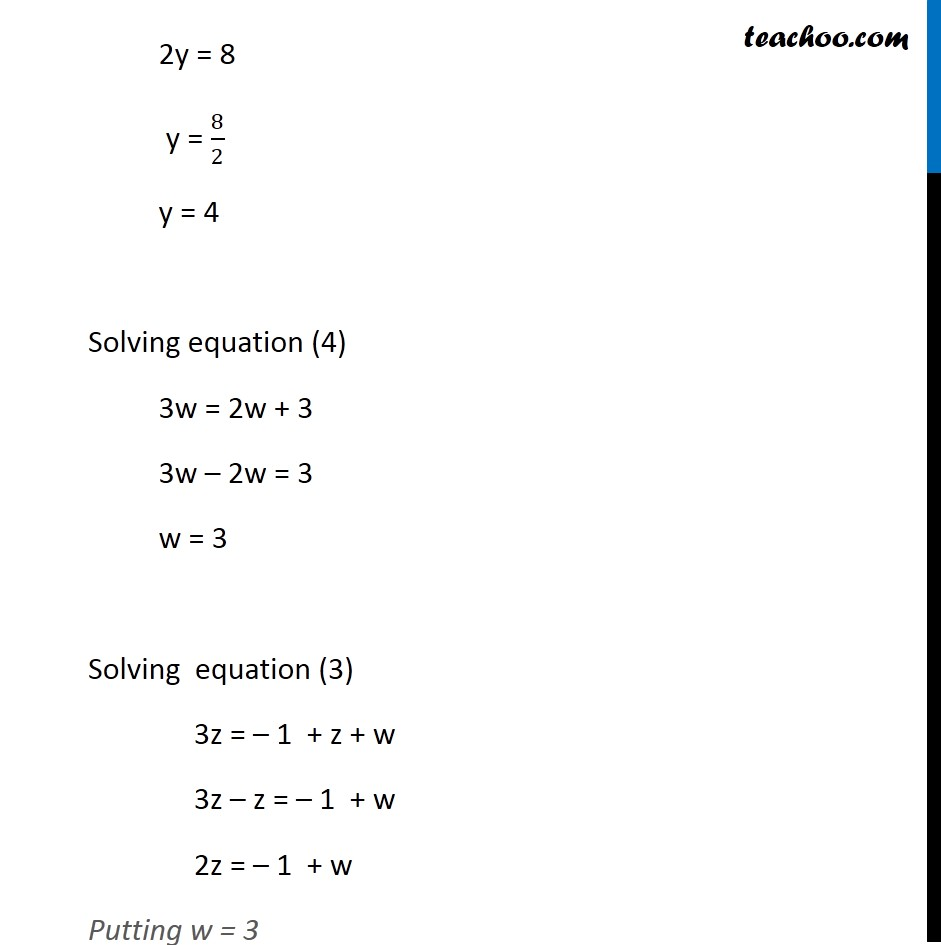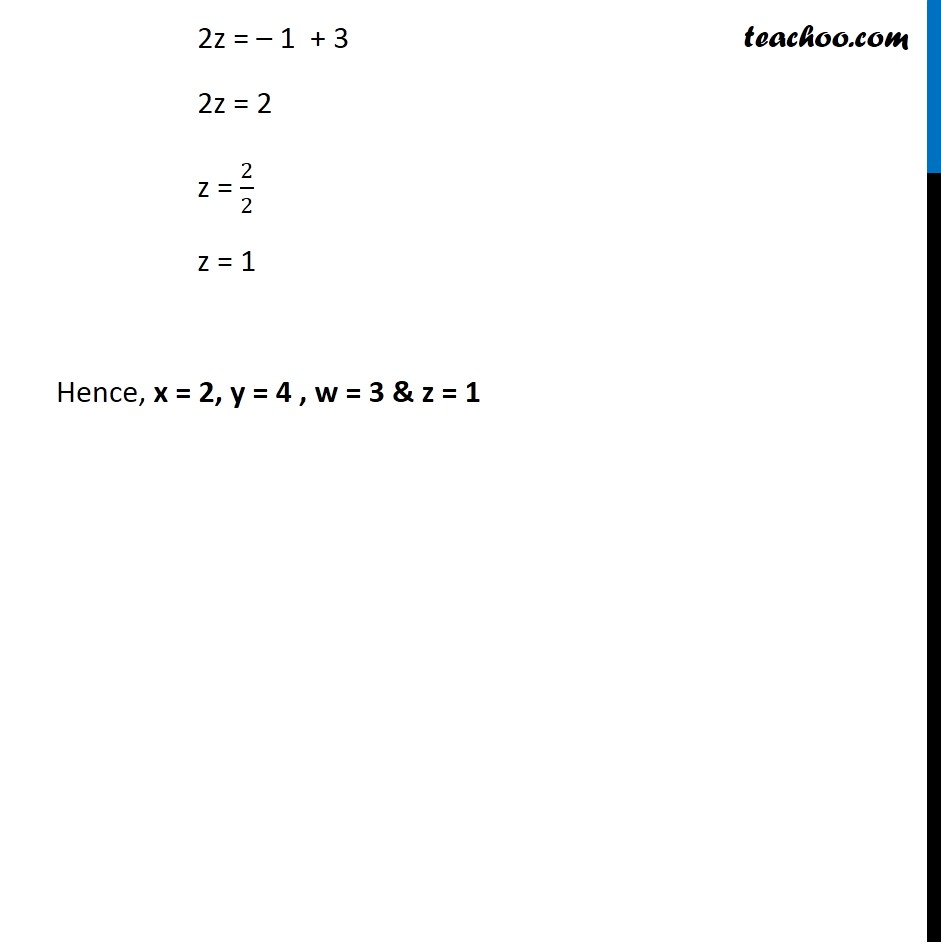1. Class 12
2. Important Questions for exams Class 12
3. Chapter 3 Class 12 Matrices

Transcript

Ex 3.2, 12 Given 3[■8(x&y@z&w)] = [■8(x&6@−1&2w)] + [■8(4&x+y@z+w&3)] find the values of x, y, z and w. 3[■8(x&y@z&w)] = [■8(x&6@−1&2w)] + [■8(4&x+y@z+w&3)] [■8(3x&3y@3z&3w)] = [■8(x+4&6+x+y@1−z+w&2w+3)] Since matrices are equal. Corresponding elements are equal 3x = x + 4 3y = 6 + x + y 3z = 1 – z + w 3w = 2w + 3 …(1) …(2) Solving equation (1) 3x = x + 4 3x – x = 4 2x = 4 x = 4/2 x = 2 Solving equation (2) 3y = 6 + x + y 3y – y = 6 + x 2y = 6 + x Putting x = 2 2y = 6 + 2 2y = 8 y = 8/2 y = 4 Solving equation (4) 3w = 2w + 3 3w – 2w = 3 w = 3 Solving equation (3) 3z = – 1 + z + w 3z – z = – 1 + w 2z = – 1 + w Putting w = 3 2z = – 1 + 3 2z = 2 z = 2/2 z = 1 Hence, x = 2, y = 4 , w = 3 & z = 1

Chapter 3 Class 12 Matrices

Class 12
Important Questions for exams Class 12